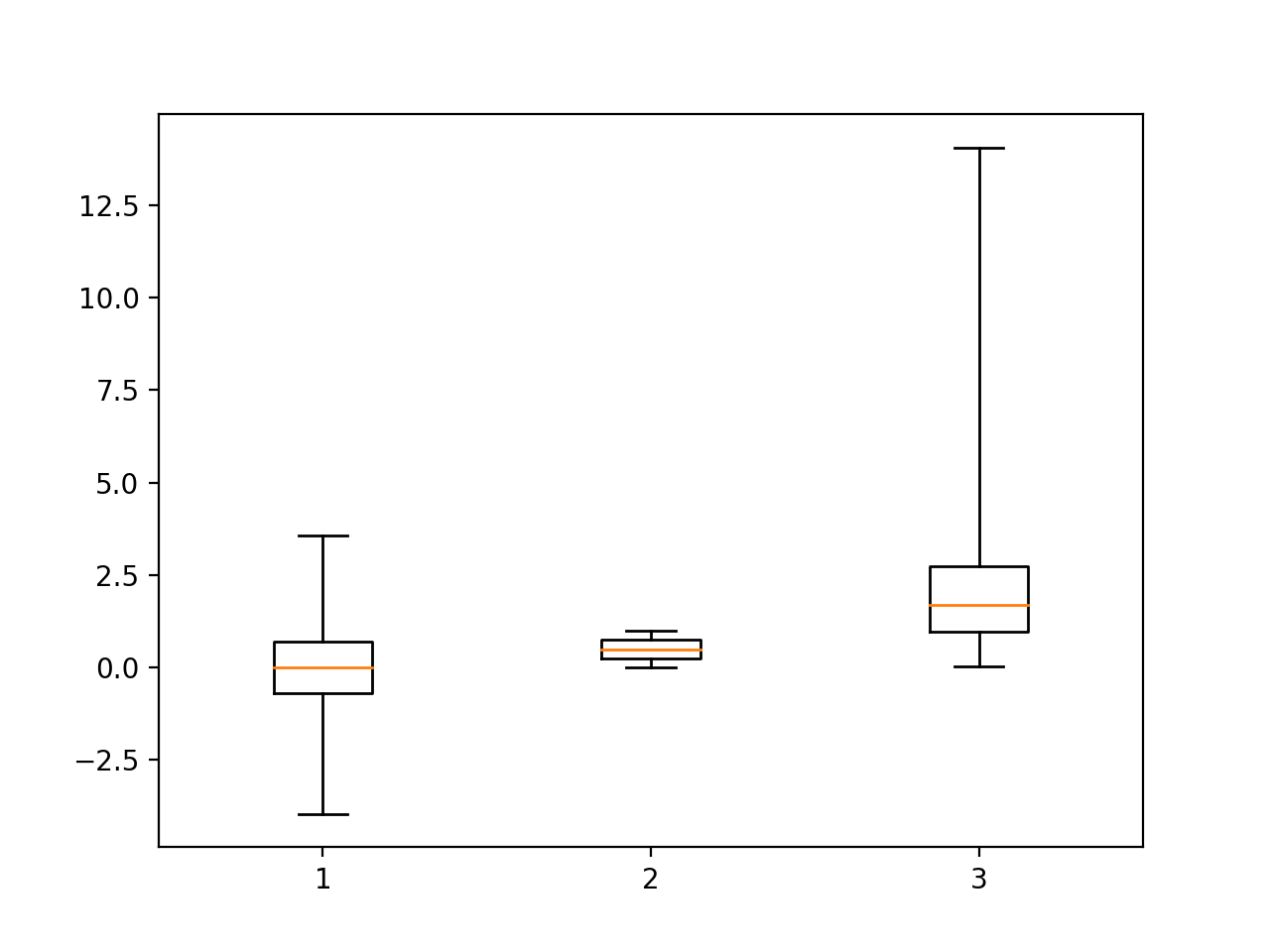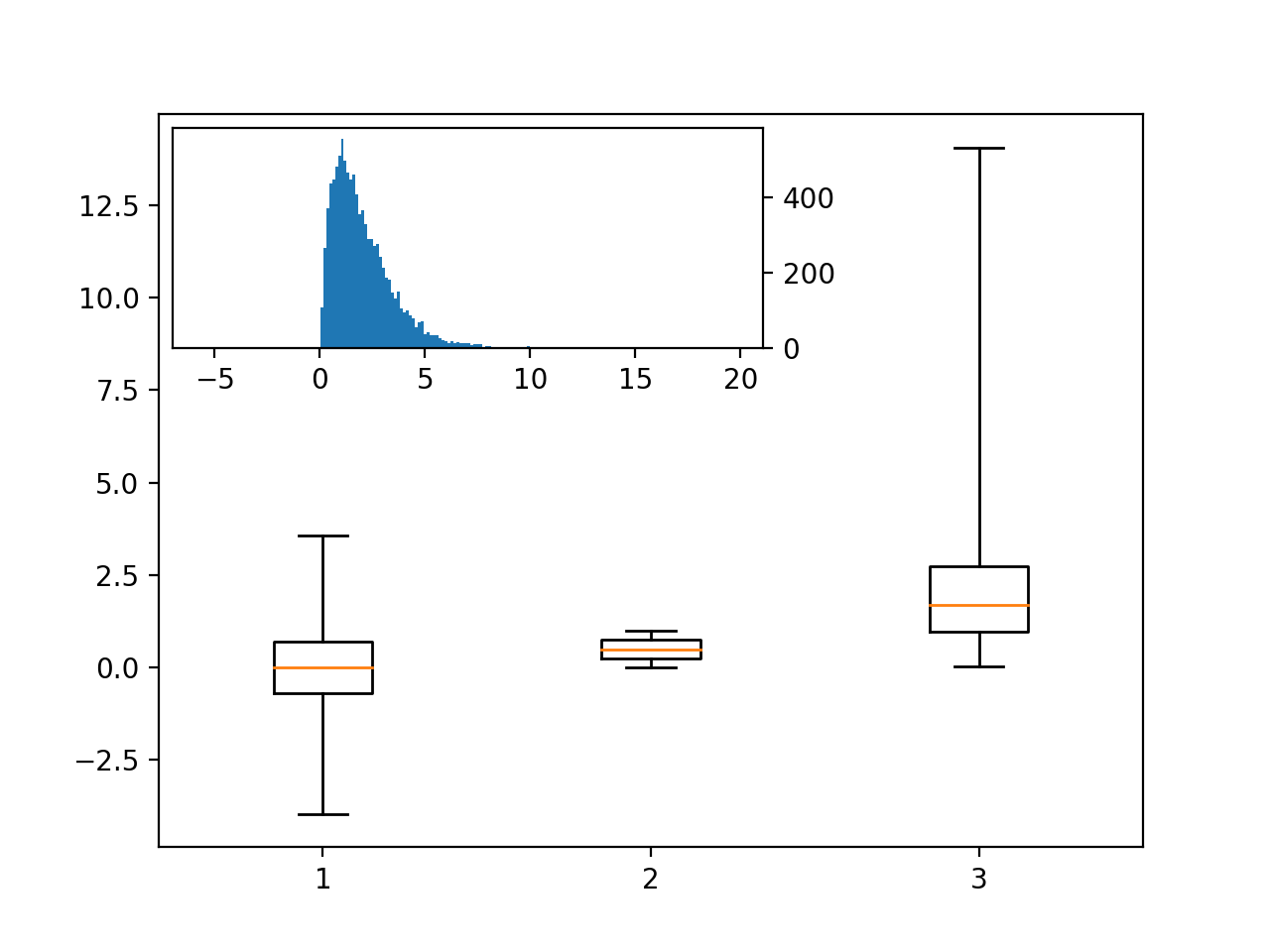# Inset Plots

We can overlay one axes on top of another within a figure, called an inset plot. This functionality is included in the matplotlib toolkits, which aren’t a “core” part of matplotlib. The toolkits do, however, tend to be included with matplotlib.

First, create the same data used for the boxplot lecture notes.

%matplotlib notebook

import matplotlib.pyplot as plt
import numpy as np
import pandas as pd

normal_sample = np.random.normal(loc=0.0, scale=1.0, size=10000)
random_sample = np.random.random(size=10000)
gamma_sample = np.random.gamma(2, size=10000)

df = pd.DataFrame({'normal': normal_sample,
'random': random_sample,
'gamma': gamma_sample})


The first step is to create the axes we want to be on the bottom in the normal way.

plt.figure()
plt.boxplot([ df['normal'],
df['random'],
df['gamma'] ],
whis='range');

<IPython.core.display.Javascript object>Then, do the same thing again, except call the inset_axes function on the mpl_il toolkit. The parameters used define the position and size of the new axis, where the size is of the percentage of the base axes. This creates the new axis.

Finally, push the new visual to this new axis, and it will behave as expected. the yaxis.tick_right() function call moves the tick marks to the right side of the inset axes.

import mpl_toolkits.axes_grid1.inset_locator as mpl_il

plt.figure()
plt.boxplot([ df['normal'],
df['random'],
df['gamma'] ],
whis='range');

ax2 = mpl_il.inset_axes(plt.gca(),
width='60%',
height='30%',
loc=2)
ax2.hist(df['gamma'],
bins=100)
ax2.margins(x=0.5)
ax2.yaxis.tick_right()

<IPython.core.display.Javascript object>